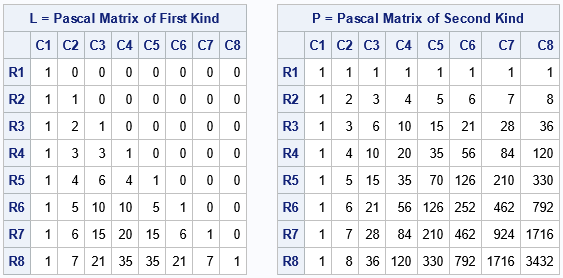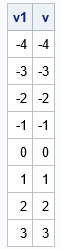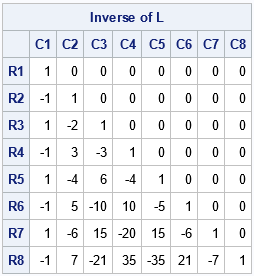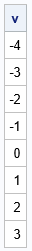Some matrices are so special that they have names. The identity matrix is the most famous, but many are named after a researcher who studied them such as the Hadamard, Hilbert, Sylvester, Toeplitz, and Vandermonde matrices. This article is about the Pascal matrix, which is formed by using elements from Pascal's triangle.

As I've previously discussed, Pascal's triangle has many interesting properties. If you put Pascal's triangle into the elements of a matrix, there are two ways you can do it. The first is to use a lower triangular matrix, L: put Pascal's triangle in the lower triangular elements and zeros in the upper triangular elements. I call this triangular matrix a Pascal matrix of the first kind. The second is to use a full matrix, P, for which the i_th antidiagonal is the i_th row of Pascal's triangle. I call this full matrix a Pascal matrix of the second kind. These two definitions are shown below for Pascal matrices that have N=8 rows:Interestingly, these two matrices are intimately connected. I have previously discussed that the full matrix (which is symmetric) has a Cholesky factorization, and that factorization is precisely P = L Lt. That's pretty cool!

### Why Cholesky factorization is important

I've written about the geometry of the Cholesky transformation and how it correlates and uncorrelates variables in statistical applications. But from the perspective of numerical linear algebra, matrix factorizations are useful because they enable us to solve certain problems efficiently. For example, if you have a symmetric matrix, P, then the Cholesky root enables you to solve the linear system P*v = b by solving two linear systems:

• Solve L w = b for the vector w.
• Solve Lt v = w for the vector v, which is also the solution to P v = b.

In the SAS IML language, you can use the TRISOLV function to solve linear systems. You use various flags to tell the TRISOLV function whether to solve a lower- or upper-triangular system, as follows:

```b = {-4, 24, 150, 528, 1452, 3432, 7293, 14300}; v1 = solve(P, b); /* general solution: solve P*v = b */   /* If you have Cholesky root (P=L*L`), you can use TRISOLV */ w = trisolv(4, L, b); /* solve L *w = b for w */ v = trisolv(3, L, w); /* solve L`*v = w for v */ print v1 v;```As the output shows, the two different ways to solve the problem give similar solutions. In fact, when you call the SOLVE function, it internally performs a decomposition and solves two triangular systems, so the two perform similar computations, although the SOLVE method does not exploit the symmetry of the Pascal matrix.

### An explicit inverse for the Pascal matrix

Some matrices have names because they have amazing mathematical properties. We've already seen that Pascal matrices (of the second kind) have Cholesky roots that are also Pascal matrices (of the first kind). But did you know that you can explicitly write down the inverse matrix for the Pascal matrices of the first kind? The following figure shows the inverse of the 8 x8 8 Pascal matrices of the first kind:The figure shows that the inverse of L looks similar to L itself except that the signs of certain elements are negative. If G is the inverse matrix, then G[i,j] = L[i,j] (-1)i + j where i=1,2,3,... is the row index and j=1,2,3,... is the column index. There are several ways to form the inverse matrix from L. The following statements create a "sign matrix," H, defined by H[i,j] = (-1)i + j. The inverse matrix for L is the elementwise multiplication of L and H, as follows:

```/* There is an EXACT inverse for L */ i = row(P); /* n x n matrix where i_th row equals i */ j = col(P); /* n x n matrix where j_th col equals j */ Sign = (-1)##(i+j); /* n x n sign matrix +/-1 */ Linv = Sign#L; print Linv[F=5. r=rname c=cname L="Inverse of L"];```

The matrix was shown earlier. You can directly solve the system P v = b by using the inverse of the Cholesky roots and two well-known results from linear algebra:

• The inverse of the product equals the (reversed) product of the inverses: inv(A*B) = inv(B)*inv(A)
• The inverse of the transpose equals the transpose of the inverse: inv(A`) = inv(A)`
Putting these results together gives a direct method to solve a linear system that involves a Pascal matrix, as follows:

```/* The inverse of the product equals the (reversed) product of the inverses. The inverse of the transpose equals the transpose of the inverse. To solve P*v = b, use v = inv(L*L`)*b = inv(L`)*inv(L)*b = (inv(L))`*inv(L)*b */ v = Linv`*Linv*b; print v;```The solution vector is identical to the solutions from other methods. However, having an explicit formula for the inverse means that you can solve a linear system that involves a Pascal matrix without performing any numerical linear algebra. For a Pascal matrix (of the second kind) You can construct the Cholesky roots without doing any linear algebra because the roots are the Pascal matrix of the first kind. Furthermore, you can explicitly construct the inverse of the Cholesky roots without doing any linear algebra. Put these facts together and you can solve linear systems that involve Pascal matrices by using only matrix multiplication.

### Summary

You might never need to solve a system of linear equations that involves a Pascal matrix, but there are some general principles that statistical programmers can learn from this article:

• If you have a matrix that has a special structure, exploit that structure. Examples include symmetric, banded, tridiagonal, and special "named" matrices. Often you can solve linear systems more efficiently if you use a specific solver rather than a general solver.
• If your matrix is a "named" matrix, read the literature to find out if there are special formulas that enable you to directly write down the form of a root or inverse matrix. Doing so can lead to an efficient way to solve linear systems.
• An interactive matrix language such as SAS/IML enables you to implement mathematical formulas in a few lines of code.

For more information about Pascal matrices, see Edelman, A. and Strang, G. (2004) "Pascal Matrices," The American Mathematical Monthly.

Share